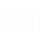In the previous blog post, we wrote about custom calculations editor in Ranet OLAP and the options it provides. Now, let’s see how to create custom calculations that can be used in pivot table report settings.

## How to create a calculated member

In order to create a calculated member, you have to follow these steps:

1. Click Add Calculated Member button on the editor's toolbar;
2. Set the custom calculation unique name:
• [Measures].[Calculated Member Name] for a calculated measure that belongs to the measures dimension;
• [Dimension].[Hierarchy].[Calculated Member Name] for a calculated member of any other dimension;
• [Dimension].[Hierarchy].[Parent name].[Calculated Member Name] for a child calculated member created for an object in the hierarchy.

If the name of the calculated member does not contain the name of the hierarchy, it belongs to the measures dimension by default.

1. Set the expression for calculating the value of the calculated member. You can use cube members, fixed numeric values, operators, MDX functions, and other custom calculations as arguments in the expression.
2. Specify the format string for the custom calculation. Choose its configuration from the list or create it manually;
3. Optional: Set the measure or a set of measures that will be used to define calculated members behavior when processing empty cells (Non-empty Behavior);
4. Optional: Set the background and foreground colors for the cell, if required.

## How to create a named set

In order to create a named set:

1. Click Add Named Set button on the toolbar;
2. Set the named set unique name;
3. Create the multidimensional expression defining the named set. It can contain OLAP cube data objects (dimensions, levels, measures, etc.), arithmetic operators, numbers, and functions.

You can create the expression:

• manually, by listing unique member names separated by commas;
• by dragging objects from the OLAP cube browser (they have to be separated by commas);
• by setting a range (the first and the last items have to be separated by a column);
• by using MDX functions that form the set by selecting the objects to include.

If the set contains groups of objects from different hierarchies, you should put the groups inside curved brackets ({}) in the expression.

## Example

Let’s review a simple example when we can use custom calculations in the pivot table.

The company offers discounts to its top 50 resellers based on their sales for the past year (in our case, the year 2008). The discount is 20% of yearly sales for resellers who sold goods for more than \$ 100,000, and 10% for resellers who have sold goods for less than \$ 100,000, but the amount of the discount cannot be higher than \$ 35,000.

For this example, we will create three custom calculations:

1.Named Set [Top 50 Resellers].

2.Two calculated members to calculate the amount of the discount: [Intermediate Discount] for calculating the discount based on the reseller’s sales and agreed discount percentage; [Final Discount] for checking if the calculated discount exceeds \$ 35,000 and setting up the final discount that can be provided to the reseller.

We hope this post was useful. If you have any questions about creating and using custom calculations in Ranet OLAP feel free to ask us.

Also, we invite you to read our blogpost about building and integration of ASP.NET pivot table.Write US